# Inequalities in One Triangle

This lesson is on Triangle Inequalities (Specifically Inequalities in One Triangle). There is a video lesson at the bottom of the post to show your class. This should give you a little extra time to get situated and back into the swing of things before Christmas Break!

## Angle Side Theorem - Inequalities in One Triangle

“If one side of a triangle is longer than another side, then the angle opposite the longer side is larger than the angle opposite the shorter side.”### Sample Problem 1:

Write the angles in order from smallest to largest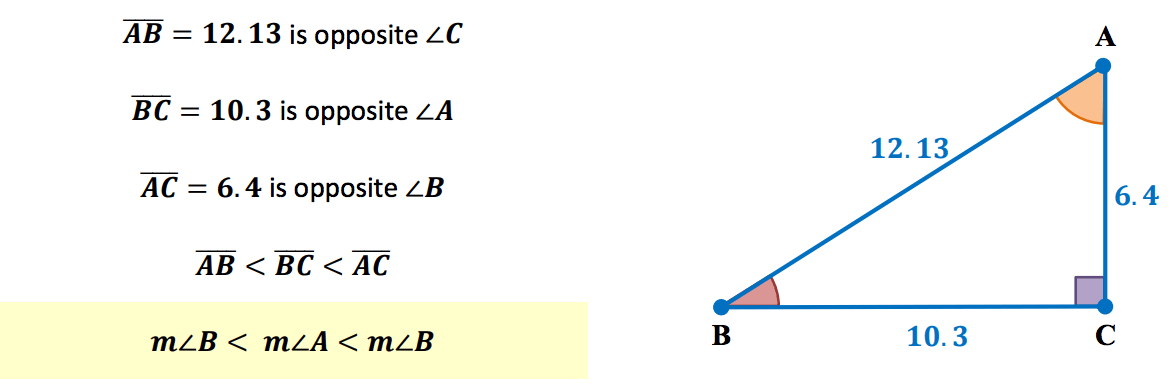## Converse of Angle Side Theorem - Inequalities in One Triangle

“If one angle of a triangle is larger than another angle, then the side opposite the larger angle is longer than the side opposite the smaller angle.”### Sample Problem 2:

Write the sides in order from shortest to longest.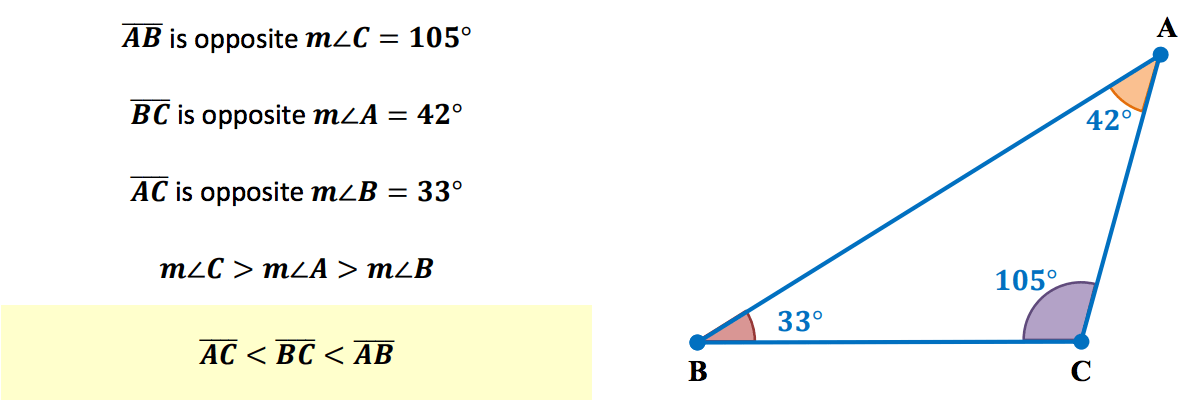## Exterior Angle Inequality Theorem

“The measure of an exterior angle of a triangle is greater than the measure of either of its remote interior angles.”### Sample Problem 3:

Determine the smallest and the largest angles.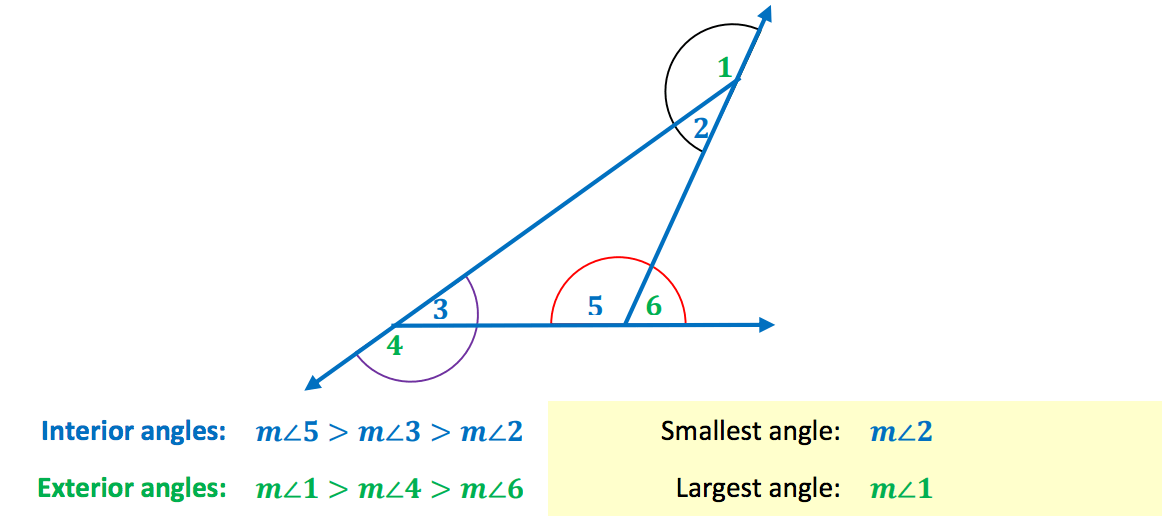## Triangle Inequality Theorem

“The sum of the lengths of any two sides of a triangle is greater than the length of the third side.”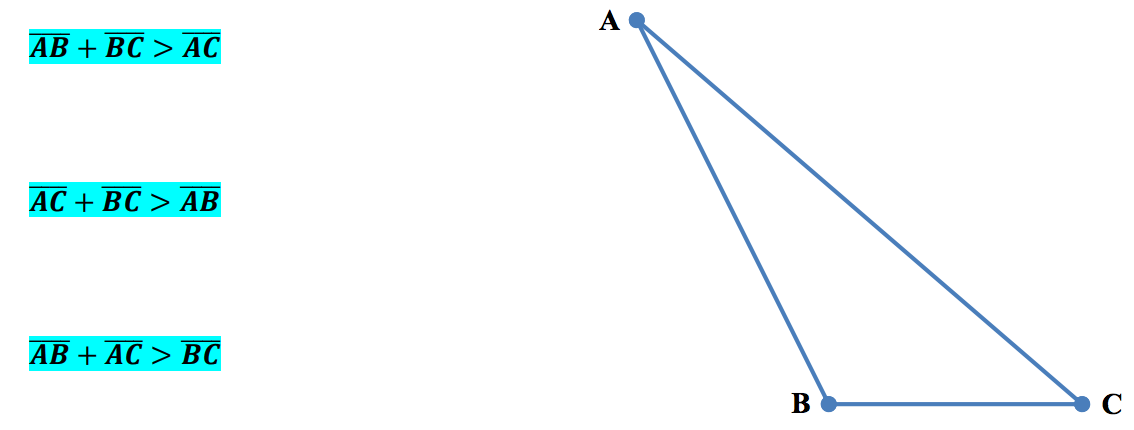### Sample Problem 4:

A triangle has one side of length 12 and another of length 8. Identify the possible lengths of the third side.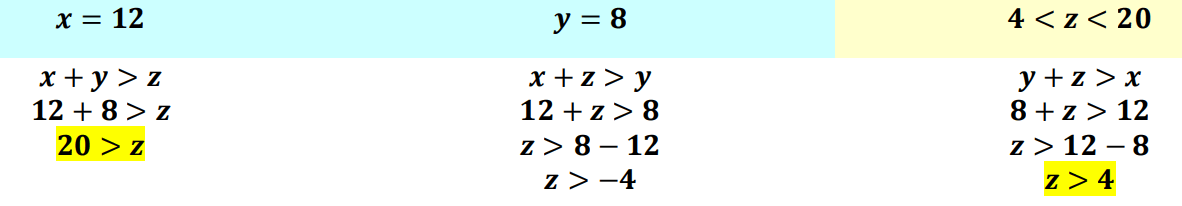## Here is your Free Content for this Lesson!

### Inequalities in One Triangle - Word Docs & PowerPoints

To gain access to our editable content Join the Geometry Teacher Community!
Here you will find hundreds of lessons, a community of teachers for support, and materials that are always up to date with the latest standards.

### Inequalities in One Triangle Worksheet - PDFs

Assignment Teacher Edition - (Members Only)

Bell Work Teacher Edition - (Members Only)

Exit Quiz Teacher Edition - (Members Only)

Guided Notes SE - ( FREE )

Lesson Plan - (Members Only)

Online Activities - (Members Only)